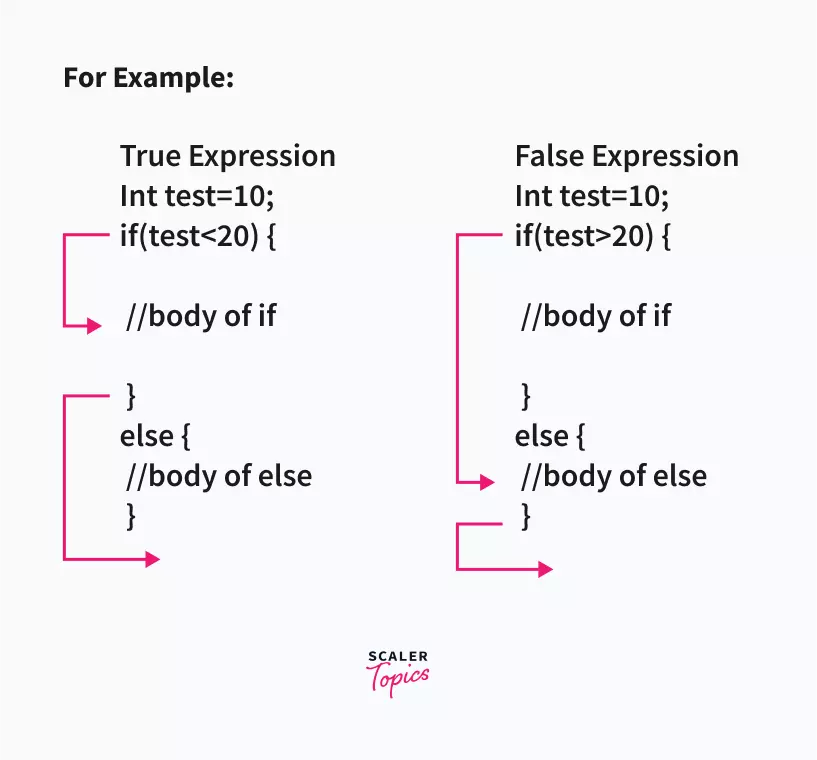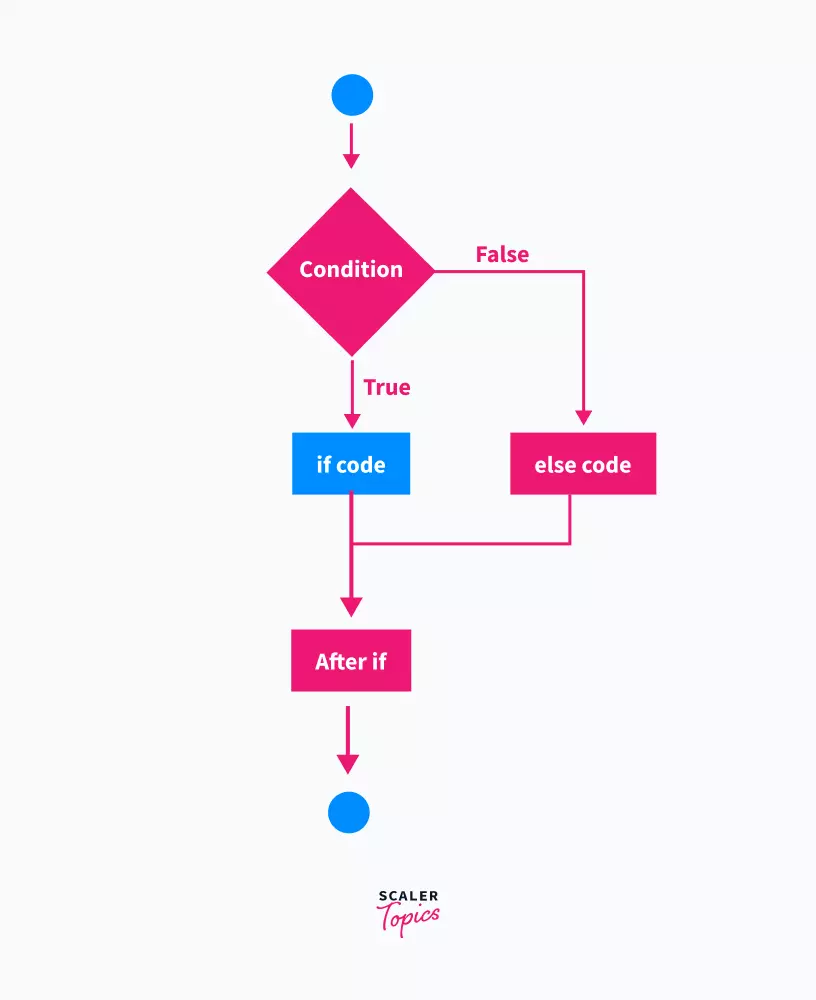# C if...else Statement

Challenge Inside! : Find out where you stand! Try quiz, solve problems & win rewards!
Learn via video courseC++ Course: Learn the Essentials
By Prateek NarangFree
5Enrolled: 1000C++ Course: Learn the Essentials
Prateek NarangFree
5
Enrolled: 1000

## Overview

The if-else statement in C programming is a fundamental control flow mechanism that allows for conditional execution of code segments. It enables a program to make decisions and execute specific sets of instructions based on the evaluation of a given condition. If the condition is true, the program executes the code inside the if block; if false, the code inside the else block is executed. This mechanism of decision-making ensures that the program responds correctly to different situations and inputs, making it a crucial tool for programmers to direct the program's flow and behavior.

## C if Statement

The if statement is used to evaluate a particular condition. If the condition is true, then the if block statement is executed else the if block is skipped and further program is executed.

## C if-else Statement

In the C programming language, the if-else statement is used for decision-making. If the given condition is true, then the code inside if block is executed, otherwise else block code is executed.

In the C programming language, any non-zero and non-null values are assumed as true, and zero or null, values are assumed as false values.

## How to use if-else in C?

Here's a simple program that checks if a number is positive, negative, or zero using the if-else statement:

Output:

For an input of 5, the output would be:

For an input of -3, the output would be:

For an input of 0, the output would be:

By following the above steps and using the provided example as a reference, you can effectively use the if-else statement in C for various decision-making scenarios in your programs.

## How if-else statement in C works?

If-else statement allows to make a decision according to the given conditions. If the given condition is true, then the statements inside the body of logical 'if' are executed and the statements inside the body of else are not executed. Similarly, if the condition is false, then the statements inside the body of ‘if’ are ignored and the statements inside the ‘else’ are executed.

For a more clear understanding of the concept, let’s take an example of xyz expression:

If the "xyz expression" is true:

• statements inside the body of if are executed
• statements inside the body of else are ignored

If the "xyz expression" is false:

• statements inside the body of if are ignored
• statements inside the body of else are executed

Basically, the if-else statement controls the flow of a program and is hence also termed as Control Flow statement.In the above example, the value of test is 10. Since, the value of test is less than 20, so the if block on LHS is executed whereas the condition is false on RHS, so the if block code is not executed and it goes to the else block.

## Flowchart of the if-else statement in C## Example of if-else statement in C

#### Program to check whether a given number is even or odd.

We provided 4 as the input number, since 4 is an even number so the condition of if statement evaluates to true and hence the if block code is executed and we get the below output.

Output

### Program to check whether a person is eligible to vote or not

We input 18 as the age which is equal to 18, hence the if condition evaluates to true and we get "You are eligible to vote" as output. Input

Output

Input

Output

## Advantages of if-else Statement in C

• if-else statement helps us to make decision in programming and execute the right code.
• It also helps in debugging of code.

## Disadvantages of if-else Statement in C

• if-else statements increase the number of code paths to be tested.
• If there are a lot of if statements the code sometimes becomes unreadable and complex, in such cases we use Switch case statement.

## FAQs

Q. Can we skip braces around the body of the if else block in C? A. Yes, we can skip the braces { } around the body of the if-else block in C when there's only a single statement inside the if or else block. However, if there are multiple statements inside the block, braces are necessary to ensure all the statements are executed as part of the condition. It's generally recommended to use braces even for single statements for better code readability and to avoid potential mistakes.

Q. What is an if-else statement example? A.

Q. What are the types of if-else statements in C? A. In C there are 3 types of if-else statements which are as follows:

1. if Statement
2. if-else Statement